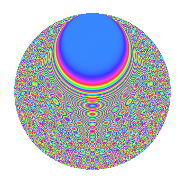# Properties

 Label 2100.2.drLevel 2100 Weight 2 Character orbit dr Rep. character $$\chi_{2100}(73,\cdot)$$ Character field $$\Q(\zeta_{60})$$ Dimension 640 Sturm bound 960

# Related objects

## Defining parameters

 Level: $$N$$ $$=$$ $$2100 = 2^{2} \cdot 3 \cdot 5^{2} \cdot 7$$ Weight: $$k$$ $$=$$ $$2$$ Character orbit: $$[\chi]$$ $$=$$ 2100.dr (of order $$60$$ and degree $$16$$) Character conductor: $$\operatorname{cond}(\chi)$$ $$=$$ $$175$$ Character field: $$\Q(\zeta_{60})$$ Sturm bound: $$960$$

## Dimensions

The following table gives the dimensions of various subspaces of $$M_{2}(2100, [\chi])$$.

Total New Old
Modular forms 7872 640 7232
Cusp forms 7488 640 6848
Eisenstein series 384 0 384

## Trace form

 $$640q + 12q^{5} + O(q^{10})$$ $$640q + 12q^{5} - 8q^{15} + 24q^{23} + 4q^{25} - 80q^{29} - 12q^{33} - 40q^{35} - 20q^{37} - 136q^{43} - 12q^{47} + 120q^{53} + 16q^{57} + 240q^{59} + 12q^{63} + 52q^{65} + 60q^{73} + 48q^{75} + 84q^{77} - 80q^{81} + 8q^{85} + 48q^{87} + 32q^{93} - 44q^{95} + O(q^{100})$$

## Decomposition of $$S_{2}^{\mathrm{new}}(2100, [\chi])$$ into newform subspaces

The newforms in this space have not yet been added to the LMFDB.

## Decomposition of $$S_{2}^{\mathrm{old}}(2100, [\chi])$$ into lower level spaces

$$S_{2}^{\mathrm{old}}(2100, [\chi]) \cong$$ $$S_{2}^{\mathrm{new}}(175, [\chi])$$$$^{\oplus 6}$$$$\oplus$$$$S_{2}^{\mathrm{new}}(350, [\chi])$$$$^{\oplus 4}$$$$\oplus$$$$S_{2}^{\mathrm{new}}(525, [\chi])$$$$^{\oplus 3}$$$$\oplus$$$$S_{2}^{\mathrm{new}}(700, [\chi])$$$$^{\oplus 2}$$$$\oplus$$$$S_{2}^{\mathrm{new}}(1050, [\chi])$$$$^{\oplus 2}$$

## Hecke characteristic polynomials

There are no characteristic polynomials of Hecke operators in the database